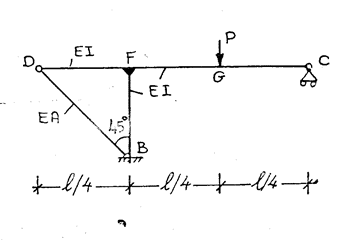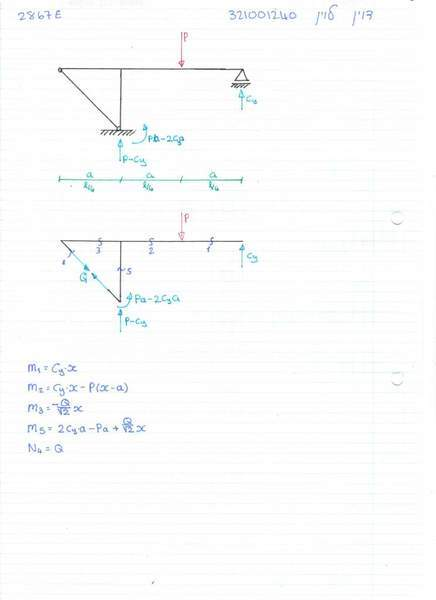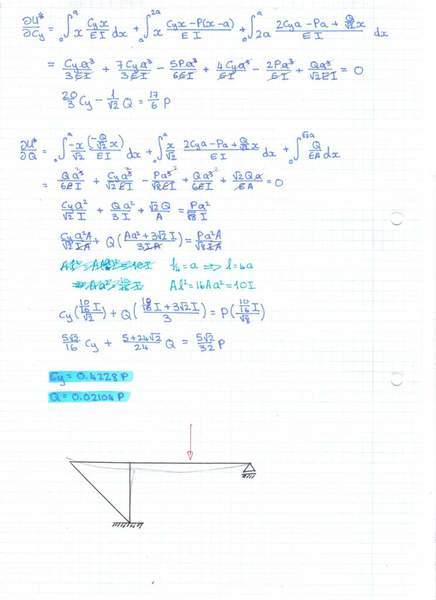# Castiglianos second theorem for indeterminate structures

Dell
in the following question it is known that the rod BD has only axial internal forces, (which i called Q) if AL2=10*I, find the reaction at C and the internal force Q.
Assume that the energy of normal forces is negligible compared to moment where there are both.instead of using L/4 i defined L/4=a

the correct answers are supposed to be Cy=0.346P and Q=0.042P

these are my calculations

i have tried solving it 4 times now and cant get the correct answer.